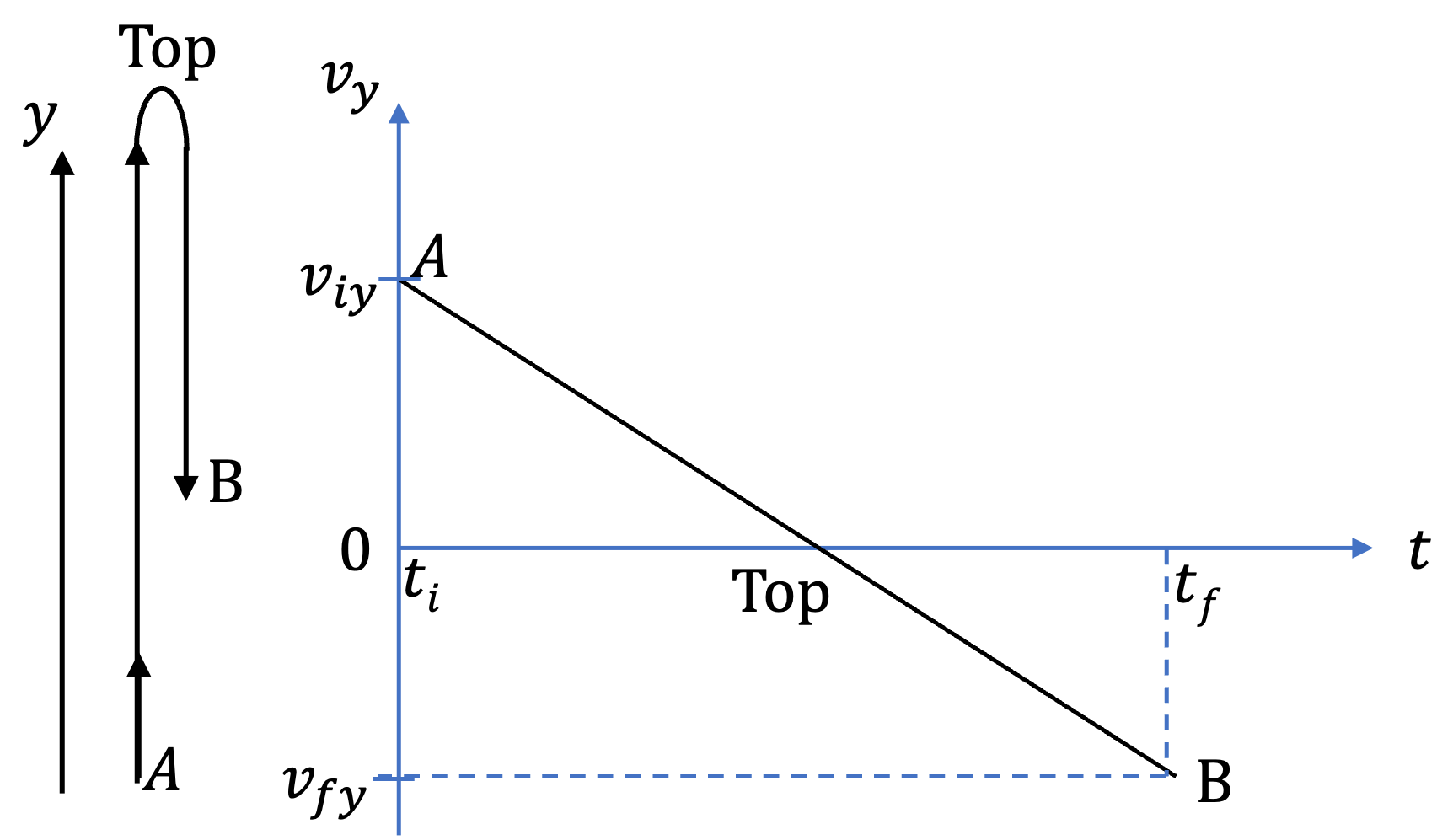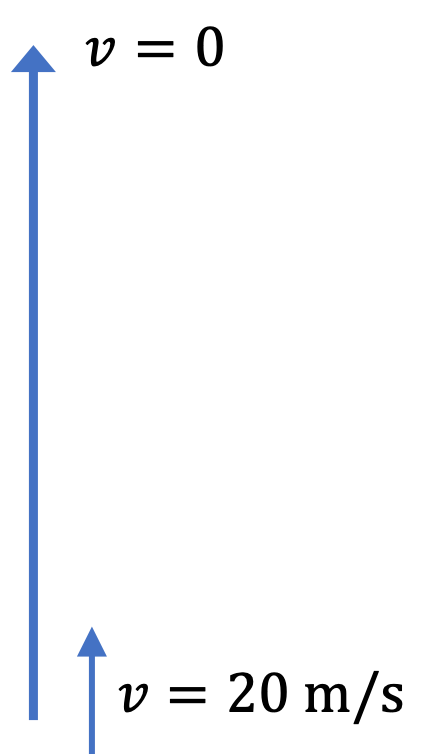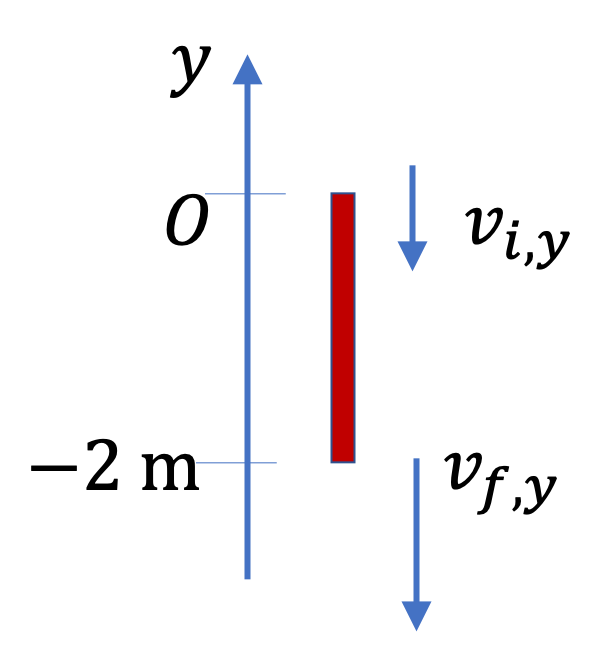## Section2.8Freely Falling Motion

The motion of freely falling objects is a special application of constant-acceleration equations. The magnitude of acceleration is denoted by letter $g\text{.}$ Near Earth, the value of $g$ is $9.81 \text{ m/s}^2$ and near Moon, it about $\frac{1}{6}\,g\text{.}$

The phrase “freely falling” refers to three types of motion: (i) falling down with increasing speed, (ii) going up as it slows down, and (iii) some part of the motion is going up and other part is falling down. When you throw something at an angle to the vertical, you get a projectile motion, which is a free fall in two-dimensions - the vertical part is free fall and the horizontal part is uniform motion with zero acceleration. We will study them in another chapter. Here, we focus on only the vertical motion.

Traditionally, we use $y$ axis pointed up for the vertical motion. Then, the acceleration will be denoted by the $y$-acceleration, denoted by $a_y \text{.}$ With positive $y$ axis pointed up, its value will be negative.

\begin{equation*} a_y = -g, \text{ or, } a_y = -9.81\text{ m/s}^2. \end{equation*}

Note that $g$ itself is positive. When someone says negative $g\text{,}$ he/she is refering to the Cartesian component $a_y$ with positive $y$ pointed up, or similarly if some other axis is pointed up. Make sure you understand how signs of velocities and acceleration in your formulas must be set otherwise you will make mistakes all the time. Figure 2.8.1 goes over logic of some of these sign considerations.

If you throw a ball vertically up, then, when the ball is on its way up, its velocity is pointed up but the acceleration is pointed down. When moving upward $v_y \gt 0$ and $a_y = -9.81\text{ m/s}^2\text{.}$ That means, the ball slows down and comes to rest for a moment when it is at the top of the trajectory. But, acceleration is still the same value. When at the top $v_y =0 0$ and $a_y = -9.81\text{ m/s}^2\text{.}$Figure 2.8.1. Ball is going up at A, momentarily stops at the Top, and then coming down at B.

After the ball has reached the maximum height and starts to come down, the velocity and acceleration both point down, i.e., $v_y \lt 0$ and $a_y = -9.81\text{ m/s}^2\text{.}$ That is why speed picks up when an object is coming down.

A ball is thrown up with speed $30.0 \text{ m/s}\text{.}$ (a) How high does the ball rise before coming down? (b) How long does it take to get there?

Hint

Think the motion on $y$ axis and try using the constant acceleration equations. If you point $+y$ axis up, then on when the ball is going up, $v_y \gt 0$ but $a_y \lt 0\text{.}$

(a) $45.9\text{ m}\text{,}$ (b) $3.06\text{ s}\text{.}$

Solution 1 (a)

You should first note that there are other ways of doing this problem than what I will give below. For instance, you could find $t$ first and then use $v_{av}$ to find $\Delta y\text{.}$

For the sake of brevity, we will drop the coordinate subscript $y$ from the symbols and use $t$ in place of $\Delta t\text{.}$ Let us use $y$ axis for the vertical motion with origin at the point the ball left and the direction of positive $y$ being up. This will make $a = -9.81\text{ m/s}^2\text{.}$(a) We are given:

\begin{equation*} v_i = 30.0 \text{ m/s}, \ \ v_f = 0, \ \ a = - 9.81 \text{ m/s}^2, \end{equation*}

and we are to find $\Delta y\text{,}$ which is another way to write $y_f-y_i\text{,}$ or height $h\text{,}$ in this case. Using the “no $t$ equation” we get (I will omit units in calculations.)

\begin{equation*} v_f^2 - v_i^2 = 2 a \Delta y \rightarrow 0 - 30^2 = 2 \times 9.81 \times \Delta y \end{equation*}

This gives

\begin{equation*} \Delta y = 45.9 \text{ m}. \end{equation*}
Solution 2 (b)

(b) We can then use $v_i, v_f, \text{ and } a$ to find $t \text{.}$

\begin{equation*} v_f - v_i = a t \rightarrow t = \dfrac{0 - 30 \text{ m/s}}{ -9.81\text{ m/s}^2} = 3.06\text{ s}. \end{equation*}

A steel ball is lauched vertically up with speed $20\text{ m/s}\text{,}$ i.e., an immediate instant after the ball leaves the launcher its speed is $20\text{ m/s}\text{.}$

(a) How long does it take to reach the top of its trajectory?

(b) How high does the ball rise before turning back?Hint

The velocity at the top is zero since velocity goes from being positive to being negative.

(a) $2.04\text{ sec} \text{,}$ (b) $40.8\text{ m}\text{.}$

Solution 1 (a)

For the sake of brevity, I will drop the coordinate subscript $y$ from the symbols. Let us use $y$ axis for the vertical motion with origin at the point the ball left and the direction of positive $y$ being up. This will make $a = -9.81\text{ m/s}^2\text{.}$

(a) The velocity along the path before reaching the top is positive, and after the ball starts to fall, the velocity is negative. That means, right at the top point, velocity is zero. We take $t_i$ the instant of launch and $t_f$ at the top. The information in the problem statement can be summarized now.

\begin{equation*} a = -9.81\text{ m/s}^2,\ \ v_i = + 20\text{ m/s},\ \ v_f = 0,\ \ \Delta t = ? \end{equation*}

We can get $\Delta t$ by using the acceleration equation.

\begin{equation*} \Delta v = a\, \Delta t \Longrightarrow \Delta t = \dfrac{\Delta v}{a}. \end{equation*}

Therefore,

\begin{equation*} \Delta t = \dfrac{0 - 20\text{ m/s}}{-9.81\text{ m/s}^2} = 2.04\text{ sec}. \end{equation*}
Solution 2 (b)

(b) Now that we know the time, we can easily get height $h$ by multiplying the average velocity by this time.

\begin{equation*} h = v_{\text{av}}\, t = \dfrac{20 + 0}{2}\times 2.04 = 40.8\text{ m}. \end{equation*}

A steel ball is shot straight up, and on its way down, it is caught at a height of $10.0$ m above the point from which it was launched. The ball is assumed to be in free fall (i.e., its acceleration is only due to acceleratioj due to gravity) after it was launched and before it was caught. The speed of launch was 25.0 m/s.

(a) How long was the ball in the flight?

(b) What was the velocity of the ball at the instant before it was caught?

Hint

Use the constant acceleration equations.

(a) $22.85 \text{ s}\text{,}$ (b) $-20.7 \text{ m/s}\text{.}$
Solution 1 (a)

(a) Let positive $y$ axis be pointed up with $y = 0$ at the height of launch. We should note here is that we do not need to find the top point since we are only concerned with points $i$ and $f$ on the trajectory. Many students forst do $i$ to the Top and then, they do from Top to $f\text{.}$ That's just waste of time.

For brevity, I will drop the subscripts $y$ from symbols and write $t$ in place of $\Delta t\text{.}$

\begin{align*} \amp y_i = 0,\ y_f = 10.0 \text{ m}, \\ \amp v_i = 25.0 \text{ m/s},\ a = -9.81 \text{ m/s}^2. \end{align*}

We can directly find $t$ by using the “no $v_f$ equation”.

\begin{equation*} y_f - y_i = v_i t + \dfrac{1}{2} a t^2. \end{equation*}

Putting the numerical values we get a quadratic equation in $t\text{.}$

\begin{equation*} 4.905\, t^2 - 25\, t + 10 = 0. \end{equation*}

This will give us two answers - one time will correspond to the time the ball was at $10.0$ m height on its way up and the longer time is the time when it was again at that place on its way down. So, we are interested in the longer time.

\begin{align*} t \amp = \dfrac{1}{2\times 4.905} \left(-(-25) \pm \sqrt{ 25^2 - 4\times 4.905\times 10 }\right)\\ \amp = \dfrac{1}{2\times 4.905} \left(25 \pm 20.7 \right)\\ \amp = 0.44 \text{ s},\ \ 4.66 \text{ s}. \end{align*}

This gives the total hang time before getting caught to be $4.66 \text{ s}\text{.}$ The other value of $t\text{,}$ $0.44 \text{ s}$ corresponds to the time when the ball was going up at that height.

Solution 2 (b)

(b) From $t \text{,}$ we can immediately get $v_f$ by using the defining equation of average acceleration $a\text{.}$

\begin{equation*} v_f = v_i + a t = 25.0 \text{ m/s} + (- 9.81 \text{ m/s}^2)\times 4.66 \text{ s} = -20.7 \text{ m/s}. \end{equation*}

Note the sign of $v_f \text{.}$ Since the ball is coming down and the positive axis is pointed up, the sign makes sense. If we had used the time $t=0.44 \text{ s}\text{,}$ which is the time when the ball was going up here, we would have got $+20.7 \text{ m/s}\text{,}$ the same speed but with the positive sign, which corresponds to the upward motion.

A rock is falling vertically on the side of a building. To fall by a window of height $2\text{ m}\text{,}$ it takes $0.25\text{ sec}\text{.}$ Assuming free fall, find the speed of the rock at the (a) top of the window and (b) bottom of the window.Hint

Use constant acceleration motion. You can use $y$ axis with origin at the top of the window and pointed up. Then, bottom of the window will have $y=-2\text{ m}\text{.}$

$v_i = 6.78\text{ m/s}$ down, $9.23\text{ m/s}$ down.

Solution 1 (a)

(a) To make sure we have correct signs, we will use a Cartesian coordinate for the motion. Let positive $y$ axis be pointed up and the origin at the top of the window. This gives us the following data, where I have stripped unncessary subscript $y$ from symbols and written $t$ in pace of $\Delta t\text{.}$

\begin{equation*} y_i = 0,\ y_f = -2\text{ m},\ a = -9.81\text{ m/s}^2,\ t = 0.25\text{ s}. \end{equation*}

From $\Delta y = v_{i} t + \dfrac{1}{2}a t^2$ gives

\begin{equation*} v_{i} = \dfrac{-2 + 4.9\times 0.25^2}{0.25} = -6.78\text{ m/s}. \end{equation*}
Solution 2 (b)

(b) The equation $v_{f} = v_{i} + a t$ gives

\begin{equation*} v_{f} = v_{i} - g\, t = -6.775 - 9.81\times 0.25 = -9.23\text{ m/s}. \end{equation*}

To get a sense of how high from the ground is the roof of a 10-story building, two friends ('A' and 'B') decide to do the following experiment. While 'A' will shoot a steel ball from the ground straigt up, 'B' will be at the roof measuring time between when the ball goes up and when it comes down as seen at the roof.

Let $v_0$ be the (known) speed with which the ball was shot and $T$ time duration between going up and coming down at the roof (measured by 'B'). (a) Find a formula that will give you height $H$ of the building based on these two pieces of information and standard value of $g\text{.}$ (b) Check your answer: for $v_0 = 70\text{ m/s}\text{,}$ $T = 8.7\text{ sec}\text{,}$ $g=9.81\text{ m/s}^2\text{,}$ $H = 34.6\text{ m}\text{.}$

Solution

No solution provided.